# 3D Scanning with ESP-32 CAM & Line Laser (Arduino / C++)

3D Scanning using Structured Light (Slit Scanning) With ESP32 CAM, Line Lazer, coded with Arduino, which communicates to Client Application.

IntermediateShowcase (no instructions)256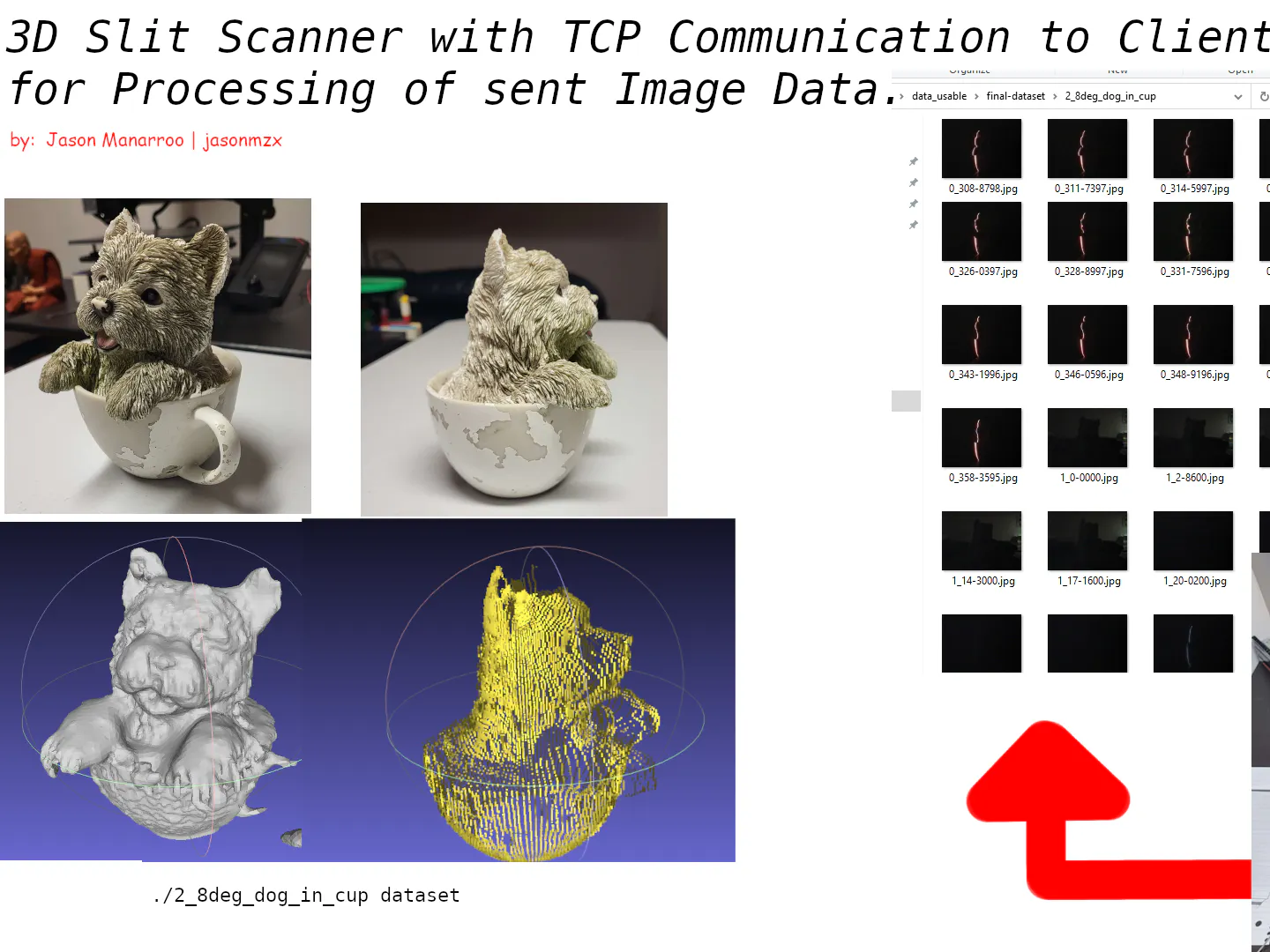## Things used in this project

### Hardware components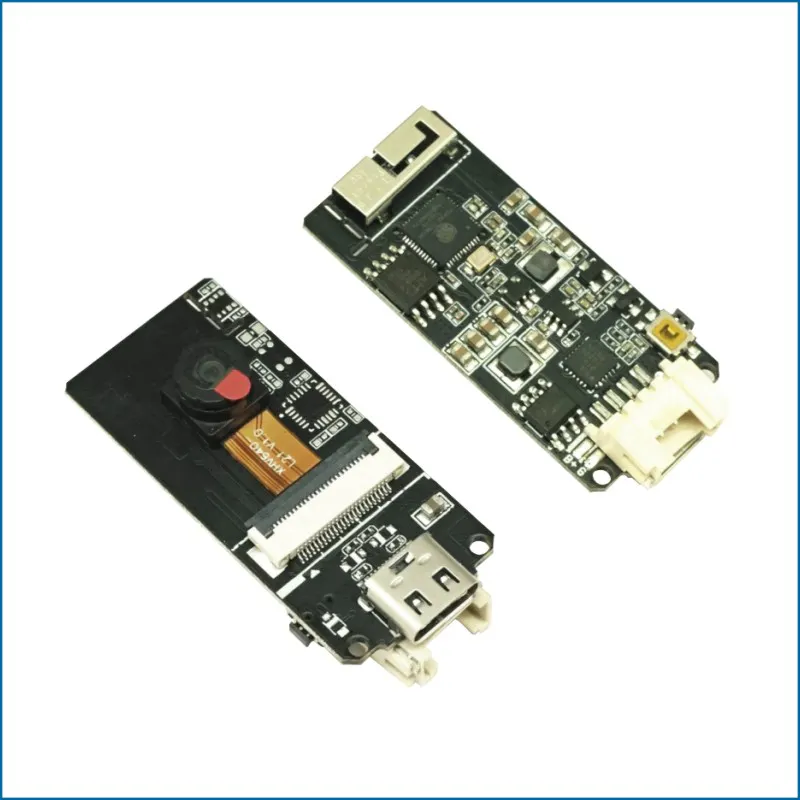M5Stack ESP32 Camera Module Development Board
×1
 Line Lazer Module (3.3V)
×1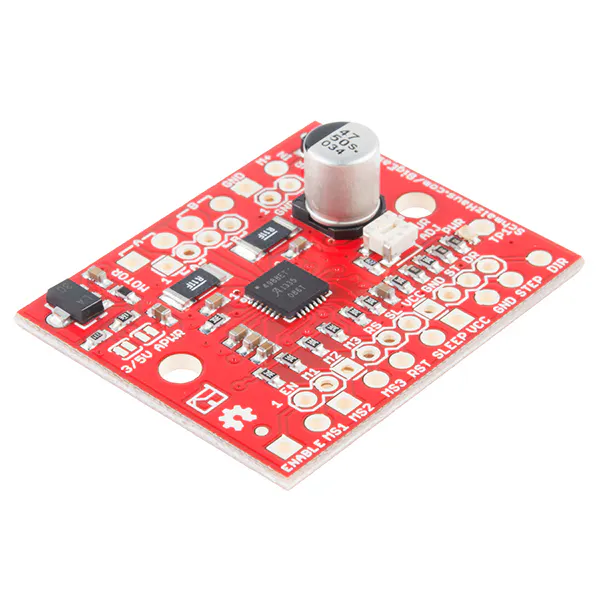SparkFun Stepper motor driver board A4988
×1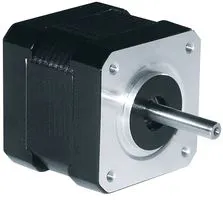Stepper Motor, Mini Step
×1

### Software apps and online servicesMicrosoft Visual Studio 2017Arduino IDE

### Hand tools and fabrication machines3D Printer (generic)

## Custom parts and enclosures

### Mechanical Document

General Specification & Documentation about how I went about the Mechanical Design (I'm a Software Engineering Major, so I can't really speak to much on it)

## Schematics

### Circuit Diagram for Hardware Setup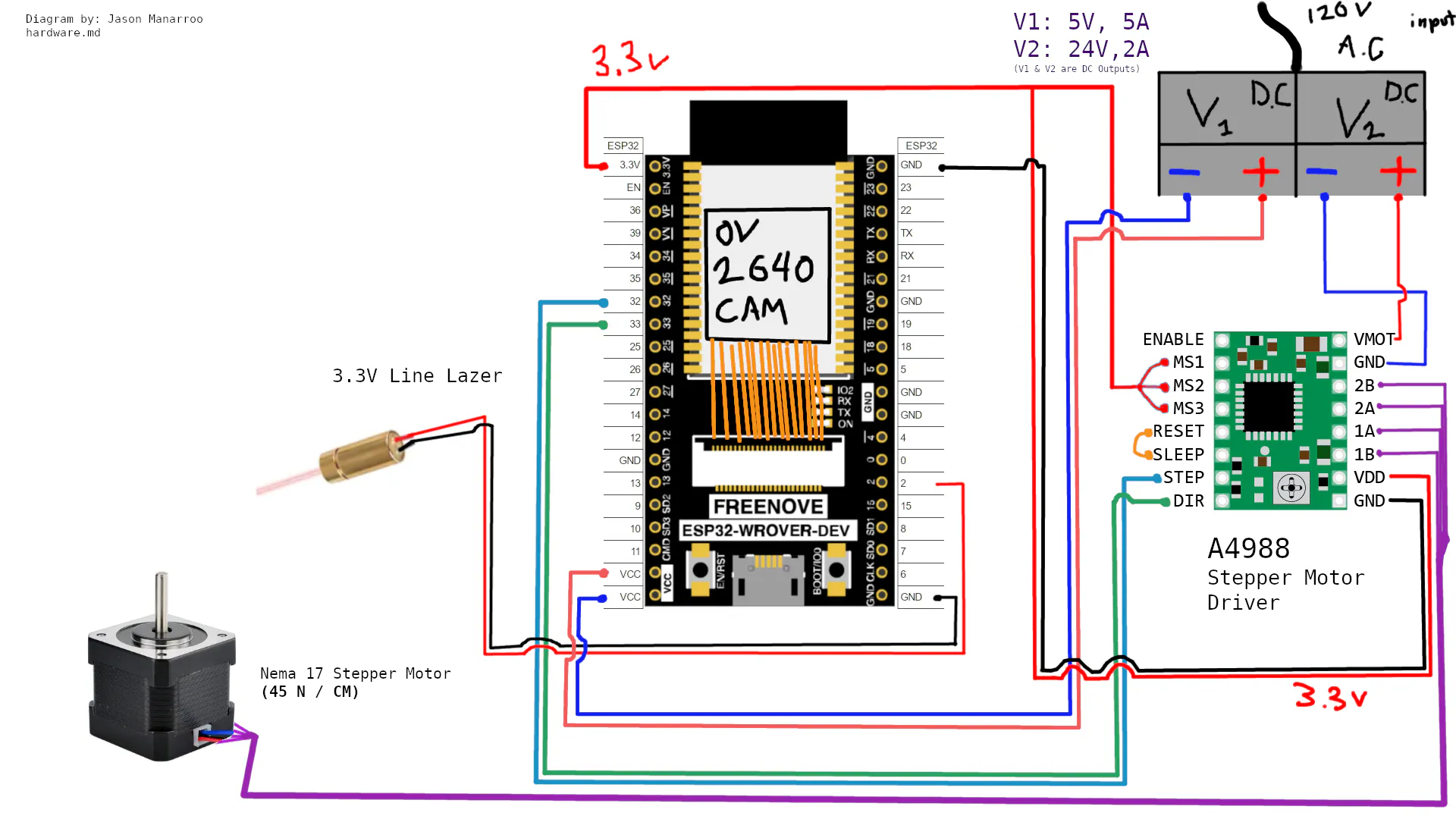## Code

### app.cpp

C/C++
```// This Project was built by: Jason Manarroo

//C++ Built-in Libraries:
#include<iostream>
#include <sstream>

// OpenGL & Related Libraries
#include<GLFW/glfw3.h>

// GLM Imports for Matrix Calculations with OpenGL
#include<glm/glm.hpp>
#include<glm/gtc/matrix_transform.hpp>
#include <glm/ext.hpp>

// OpenCV & Image Processing Libraries
#include <opencv2/imgcodecs.hpp>
#include <opencv2/highgui.hpp>
#include <opencv2/imgproc.hpp>

void tcp_server_main();

// Project Core Imports:
#include "pipeline.h"
#include "io_handle.h"
#include "camera_calibration.h"
#include "console_printing.h"

//	OpenGL Classes Imports:
#include "VAO.h"
#include "VBO.h"
#include "EBO.h"
#include "Camera.h"

// CLI COMMANDS

struct RunTcpCommand {
bool isPortSet;
int port;
};

struct RenderCommand {
std::string directory;

bool isMidpointSet;
int midpoint;

bool isCutoffSet;
int cutoff;

bool isPipelineSet;
std::string pipeline;
};

struct CameraCalibrationCommand {
std::string directory;
};

//CLI helpers

bool is_integer(const std::string& s) {
return !s.empty() && std::all_of(s.begin(), s.end(), ::isdigit);
}

template<typename T>
void cin_input_wrapper(T& var, const std::string& prompt, bool isMandatory) {
while (true) {
cinPromptMessage(prompt, isMandatory);

std::string inputLine;
std::getline(std::cin, inputLine);

// Check if inputLine contains any space(s)
if (inputLine.find(' ') != std::string::npos) {
std::string e = "Invalid input. Spaces are not allowed. Please try again...\n";
print_error(e);
continue;
}

std::stringstream ss(inputLine);

// Try to read a T from the stringstream, and ensure that there are no other characters
if (!(ss >> var) || !ss.eof()) {

if (!isMandatory) { //If the Optional isn't Mandatory, can be escaped
std::cout << bright_green << "OK, SKIPPING Optional Parameter...\n\n" << std::endl;
break;
}
std::string e = "Invalid input... Please try again...\n";
print_error(e);
}
else {
std::cout << green << "OK, Recorded !\n\n" << reset << std::endl;
std::cout << "Extracted value: " << var << std::endl;
break;
}
}
}

//Constants

const unsigned int width = 1000;
const unsigned int height = 1000;

//Loading in the Processed Object into an OpenGL Window to be displayed

std::cout << "Indices (First 20): " << std::endl;

for (int i = 0; i < 20; i++) {
std::cout << indices[i] << ", ";
}
std::cout << std::endl;

// Initialize GLFW

glfwInit();

// Tell GLFW what version of OpenGL we are using
// In this case we are using OpenGL 3.3
glfwWindowHint(GLFW_CONTEXT_VERSION_MAJOR, 3);
glfwWindowHint(GLFW_CONTEXT_VERSION_MINOR, 3);
// Tell GLFW we are using the CORE profile
// So that means we only have the modern functions
glfwWindowHint(GLFW_OPENGL_PROFILE, GLFW_OPENGL_CORE_PROFILE);

// Create a GLFWwindow object of 800 by 800 pixels, naming it "YoutubeOpenGL"
GLFWwindow* window = glfwCreateWindow(width, height, "3D Object Scanner", NULL, NULL);
// Error check if the window fails to create
if (window == NULL)
{
std::cout << "Failed to create GLFW window" << std::endl;
glfwTerminate();
return -1;
}
// Introduce the window into the current context
glfwMakeContextCurrent(window);

// Specify the viewport of OpenGL in the Window
// In this case the viewport goes from x = 0, y = 0, to x = 800, y = 800
glViewport(0, 0, width, height);

// Generates Vertex Array Object and binds it
VAO VAO1;
VAO1.Bind();

std::cout << "Vertice Size: " << vertices_size << "\n Indices Size: " << indices_size << std::endl;

// Generates Vertex Buffer Object and links it to vertices
VBO VBO1(vertices, vertices_size);
// Generates Element Buffer Object and links it to indices
EBO EBO1(indices, indices_size);

// Links VBO attributes such as coordinates and colors to VAO
VAO1.LinkAttrib(VBO1, 0, 3, GL_FLOAT, 6 * sizeof(float), (void*)0);
VAO1.LinkAttrib(VBO1, 1, 3, GL_FLOAT, 6 * sizeof(float), (void*)(3 * sizeof(float)));
//VAO1.LinkAttrib(VBO1, 2, 2, GL_FLOAT, 8 * sizeof(float), (void*)(6 * sizeof(float)));
// Unbind all to prevent accidentally modifying them
VAO1.Unbind();
VBO1.Unbind();
EBO1.Unbind();

// Gets ID of uniform called "scale"

// Original code from the tutorial
/*Texture brickTex("brick.png", GL_TEXTURE_2D, GL_TEXTURE0, GL_RGBA, GL_UNSIGNED_BYTE);

// Variables that help the rotation of the pyramid
float rotation = 0.05f;
double prevTime = glfwGetTime();

// Enables the Depth Buffer
glEnable(GL_DEPTH_TEST);

Camera camera(width, height, glm::vec3(0.0f, 0.0f, 2.0f));

// Main while loop
while (!glfwWindowShouldClose(window))
{
// Specify the color of the background
glClearColor(0.07f, 0.13f, 0.17f, 1.0f);
// Clean the back buffer and depth buffer
glClear(GL_COLOR_BUFFER_BIT | GL_DEPTH_BUFFER_BIT);
// Tell OpenGL which Shader Program we want to use

// Handles camera inputs
camera.Inputs(window);

// Assigns a value to the uniform; NOTE: Must always be done after activating the Shader Program
glUniform1f(uniID, 0.5f);
// Binds texture so that is appears in rendering
// Bind the VAO so OpenGL knows to use it
VAO1.Bind();
// Draw primitives, number of indices, datatype of indices, index of indices
glDrawElements(GL_TRIANGLES, indices_size, GL_UNSIGNED_INT, 0);
// Swap the back buffer with the front buffer
glfwSwapBuffers(window);
// Take care of all GLFW events
glfwPollEvents();
}

// Delete all the objects we've created
VAO1.Delete();
VBO1.Delete();
EBO1.Delete();
// Delete window before ending the program
glfwDestroyWindow(window);
// Terminate GLFW before ending the program
glfwTerminate();
return 0;
}

int main() {
programCredit();	//Roll the credits at start of Application

while (true) {
std::cout << "Enter command: ";
std::string command;
getline(std::cin, command);
std::istringstream iss(command);
std::vector<std::string> tokens{ std::istream_iterator<std::string>{iss},
std::istream_iterator<std::string>{} };

if (!tokens.empty()) {

// ! COMMAND EXECUTIONS HERE :

if (tokens == "rt" || tokens == "rtcp") {
RunTcpCommand runTcpCommand;
runTcpCommand.isPortSet = false;

if (tokens.size() > 1 && is_integer(tokens)) {
runTcpCommand.port = std::stoi(tokens);
runTcpCommand.isPortSet = true;
}
// Print command data or handle runTcpCommand
if (runTcpCommand.isPortSet) {
std::cout << "TCP command activated. Port: " << runTcpCommand.port << "\n";
}
else {
std::cout << "TCP command activated. No port specified.\n";
tcp_server_main();
}
}
else if (tokens == "cc" || tokens == "camera-calib") {

//Assert for Correct Command Size:
if (tokens.size() < 2) {
std::string e ="Camera Calibration Command requires a directory path.";
print_error(e);
}
else {

CameraCalibrationCommand CCComand;
CCComand.directory = tokens;

calibrate_camera_from_directory(CCComand.directory, 11, 7, true);
}

}
else if (tokens == "rc" || tokens == "r") {

if (tokens.size() < 4) {

std::string config_filepath = tokens;

if (tokens.size() == 3) {
config_filepath = default_config_path + '\\' + config_filepath;
}

//& -------------------- CONFIG FILE PRINTING --------------------
std::cout << bright_yellow << "Rendering Configuration...\n `" << tokens << "`\n\n ######### BASIC Configuration Info: #########" << reset << std::endl;

std::cout << "Directory : " << loaded_config.directory << std::endl;
std::cout << "Title : " << loaded_config.config_title << std::endl;
std::cout << "Step Angle Interval : " << loaded_config.step_angle_interval << std::endl;
std::cout << "Y_Scale : " << loaded_config.y_stretch << std::endl;

std::cout << "\nCYLINDRICAL Method EXCLUSIVE PARAMETERS:" << std::endl;
std::cout << "Lazer Angle Relative to Camera : " << loaded_config.lazer_angle_relative_2_cam << std::endl;
std::cout << "Pixel Midpoint X : " << loaded_config.pixel_midpoint_x << std::endl;

std::cout << "\nPLANAR EQUATION Method EXCLUSIVE PARAMETERS:" << std::endl;
std::cout << "PE A : " << loaded_config.pe_A << std::endl;
std::cout << "PE B : " << loaded_config.pe_B << std::endl;
std::cout << "PE C : " << loaded_config.pe_C << std::endl;
std::cout << "Translation Vector (tvec) : [" << loaded_config.translation_vector << ", " << loaded_config.translation_vector << ", " << loaded_config.translation_vector << "]" << std::endl;

std::cout << "\nExtra Parameters:" << std::endl;
std::cout << "Top Cutoff : " << loaded_config.top_cutoff << std::endl;
std::cout << "Bottom Cutoff : " << loaded_config.bottom_cutoff << std::endl;
std::cout << std::endl;
//& -------------------- CONFIG FILE PRINTING --------------------

int ogl_inst = spawnOpenGL(pipeline_response);
return 0;
}
else {
std::string e = "Couldn't find Configuration `" + tokens + "` ... Try again?";
print_error(e);
}
}
}
// This Command will be more of a "Fill in the Blanks" Kind of CLI

else if (tokens == "mc" || tokens == "mkcfg" || tokens == "mkconf") {

//Ensure the Command is called (Without Args)
if (tokens.size() < 2) {

DatasetConfig configCommand;
configCommand.is_found = true;

//! Mandatory {arams
cin_input_wrapper(configCommand.directory,
"Directory of the Dataset: (string)", 1);
cin_input_wrapper(configCommand.config_title,
"Title of this Configuration: (string)", 1);
cin_input_wrapper(configCommand.step_angle_interval,
"Step Angle Interval (EX: Rotates 2.86 Degrees Per Step) >> Answer in Degrees: (float)", 1);
"Manual Correction Value for Step Angle Interval, If you don't wish to correct | default: `1.0` : (float)", 1);
cin_input_wrapper(configCommand.y_stretch, "Y Stretch | default: `1.0` : (float)", 1);

//! Cylindrical Method
cin_input_wrapper(configCommand.lazer_angle_relative_2_cam,
"Angle of the Lazer Relative to the ESP Camera >> Answer in Degrees: (float)", 0);
cin_input_wrapper(configCommand.pixel_midpoint_x,
"X-Midpoint | If unsure, use ~200: (int)", 0);

//! Planar Equations Method
configCommand.pe_A = 0;

cin_input_wrapper(configCommand.pe_A,
"Enter `A` of Planar Equation: z = (A)*x + B*y + C | (double)", 0);

// Make sure that if the User Starts filling in the Planar Equation, they fill in everything...
if (configCommand.pe_A != 0) {
std::cout << magenta << "Since you've Entered A, you must Fill in B & C....\n\n" << reset << std::endl;
cin_input_wrapper(configCommand.pe_B,
"Enter `B` of Planar Equation: z = A*x + (B)*y + C | (double)", 1);
cin_input_wrapper(configCommand.pe_C,
"Enter `C` of Planar Equation: z = A*x + B*y + (C) | (double)", 1);
}
else {
std::cout << bright_magenta << "SKIPPING PLANAR EQ...\n" << reset << std::endl;
}

//* Getting TVEC 3

for (int i = 0; i < 3; ++i) {
float temp = 0.0;

std::string st = "Enter Translation Vector's TVEC[ " + std::to_string(i) + " ]";

cin_input_wrapper(temp, st, 0);

//If temp is still 0 on first iteration, skip everything else, the User doesn't want TVEC input
if (i == 0 && temp == 0) { //! Set TVEC to ( 0 , 0 , 0 )
configCommand.translation_vector[i] = temp;
configCommand.translation_vector[i+1] = temp;
configCommand.translation_vector[i+2] = temp;
std::cout << bright_magenta << "SKIPPING TVEC... [0,0,0]\n\n" << reset << std::endl;
break;
}

configCommand.translation_vector[i] = temp;
}

std::cout << reset << "Completed Configuration Registration... Before saving the file:" << std::endl;
std::string cfname;
std::cout << green << "Enter a filename for your Config File : <config name>.cfg" << reset << std::endl;
std::cin >> cfname;

//! Check if USER enters a config name with file extension already, If so, don't add extension to str.
// The string is either too short to contain ".cfg", or it does not end with ".cfg" therefore add it
if (cfname.length() < 4 || cfname.rfind(".cfg") != cfname.length() - 4) { cfname += ".cfg"; }

WriteConfigToFile(configCommand, cfname, std::string("configs"));
std::cout << reset << "SUCCESSFULLY SAVED CONFIG!" << std::endl;
}
}

//TODO: Implement `h` , `help` command

else {
std::string unknown_err = "Unknown Command : `" + tokens + "` ... use the `h` or `help` command for help.";
print_error(unknown_err);
}
}
}
return 0;
}
```